# Search file and create backup according to creation or modification date

1
947072Computer users often have a problem with file search as they tend to forget the location or path of a file even though Windows provides a file search utility. Sometimes we copy the files from a pen drive or save the file from the Internet after saving the files we forget the location even we don’t remember the name of the file. It can be very difficult to find out the file which has been saved 2 days ago without knowing the name.  In this article, I am going to discuss the program which will help you to find the file.

Let us first understand the program’s logic. Figure 1 explains this. Let us first do indexing or, in Python language terms, let’s construct a dictionary in which the file with the path will be the key of the dictionary and the value will be the tuple which contains creation date and modification date of the file. The dictionary will be dumped into the pickle file. The next time, the file will be searched in the dictionary (dumped in the pickle file).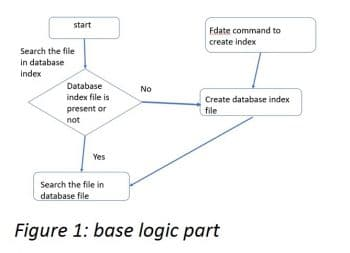Now that you have understood the logic of the program, let us look at the program in detail. I have broken it into different functions and methods. Let’s see what each function and method do. The program name is fdate.py

The block of code below imports the essential modules:

import os

import cPickle

import argparse

from collections import OrderedDict

from datetime import datetime, timedelta

import time

import shutil

from colorama import init,Fore, Back, Style

import urllib

import re

import subprocess

import webbrowser

init(autoreset=True)

• Create a class file_backup which hold the all the methods.
```class file_backup():

dict1 = {}

def __init__(self):

pass```
• The below method is responsible for version update. It will check the current version of the program on my website. If your version is old then it would give you the message.
```def version_get(self,version1):

url2 = 'http://l4wisdom.com/fdate.php'

try:

http_r = urllib.urlopen(url2)

for each in http_r:

if 'Current Version' in each:

cur_version = float(each.split('=').strip("\n"))

if cur_version>version1:

except :

pass```
• The below method would return the list of drives in your Windows.
```def get_drives(self):

response = os.popen("wmic logicaldisk get caption")

list1 = []

line = line.strip("\n")

line = line.strip("\r")

line = line.strip(" ")

if (line == "Caption" or line == ""):

continue

list1.append(line)

return list1```
• The below method opens the index database file and load the dictionary into memory.
```def file_open(self):

pickle_file = open("fdate.raj", "r")

pickle_file.close()

return file_dict```
• The below method stores the created dictionary into disk.
```def strore_files(self):

pickle_file = open("fdate.raj", "w")

cPickle.dump(file_backup.dict1, pickle_file)

pickle_file.close()```
• The below method is responsible to create the dictionary. The dictionary which contains key as a file name with its path and value as a tuple which contains creation and modification date of the file.
```def search1(self, drive):

file_t= open("Error_fdate.txt",'w')

for root, dir, files in os.walk(drive, topdown=True):

for file in files:

file = file.lower()

file_path = root+'\\'+file

#print file_path

try:

creation_time = os.path.getctime(file_path)

modification_time = os.path.getmtime(file_path)

tuple1 = (creation_time,modification_time)

file_backup.dict1[file_path] = tuple1

except Exception as e :

str1 = str(e)+'\n'

file_t.write(str1)

file_t.close()```
• The below method performs the copy or move operations per user’s need.
```def copy_files(self, path_to_copy, files,m_or_c=0 ):

try:

for file in files:

if m_or_c==0:

shutil.copy(file,path_to_copy)

print file," Copied"

else :

shutil.move(file, path_to_copy)

print file, " Moved"

except Exception as e :

print e```
• The below method filters the output according to matching string provided with -m option by the user.
```@staticmethod

def matching_string(match1, all_file):

maching_files = {}

for k,v in all_file.iteritems():

if re.search(match1,k):

maching_files[k]=v

return maching_files```
• The below method removes the entries from the output according to matching string provided with -e option by the user.
```@staticmethod

def filter_list(e_list,all_files):

for each in all_files.keys():

each1= each.lower()

for e in e_list:

e = e.lower()

if each1.startswith(e):

del all_files[each]

return all_files```
• The below method runs the selected file given by user.
```@staticmethod

def file_run(path1):

path = path1[2:]

file_list = path.split("\\")

os.chdir(path1[0:2] + '//')

file_to_open = file_list[-1]

file_list= file_list[:-1]

if file_list:

for each in file_list:

os.chdir(each)

file_name = '"' + file_to_open + '"'

os.startfile(file_name)```
• The below method opens the folder of the selected file.
```@staticmethod

def folder_open(path):

print "path*****", path

path = path.rsplit("\\", 1)

path_list = path.split("\\")

# print path_list

os.chdir(path_list[0:2])

if path_list[2:]:

os.chdir(path_list[2:])

if len(path_list) > 1:

for i in xrange(1, len(path_list) - 1):

os.chdir(path_list[i])

os.chdir(path_list[-1].split(":"))

subprocess.Popen(r'explorer /select,"." ')```
• The below method is responsible to display the files according to the creation or modification date provided by the user.
```   def file_get(self,time_stamp,latest=None,m=0, order= False,
number_of_files=0,e_list=None,match=None):

a = (Fore.RED + '------------------------------------------------------')

b = (Fore.BLUE + '|')

t1 = datetime.now()

i = 0

d1 = {}

all_file = self.file_open()

if not latest:

latest = time.time()

if e_list: #Exception list

all_file=self.filter_list(e_list,all_file)

if match:

all_file= self.matching_string(match,all_file)

for key,values in all_file.iteritems():

c_date = values[m]

if c_date>= time_stamp and c_date<= latest:

d1[key]= c_date

d2 = OrderedDict(sorted(d1.items(), key=lambda (k, v): v, reverse=order))

for k,v in d2.iteritems():

i = i + 1

print i, ": ", k, b ,time.strftime("%a, %d %b %Y %H:%M:%S ", time.localtime(v))

print a

if i== number_of_files:

break

print "Total files are ", i

t2 = datetime.now()

total_time = t2 - t1

print "Time taken ", total_time

try:

if i:```
• The following code asks the options whether to open, move or copy the files.
```ch = raw_input("Press D to open folder or F to open file or M to move files
or C to copy files\t")

ch = ch.lower()

if ch == 'c' or ch=='m':

n = raw_input('Enter the number of the files for multiple files
give number with space or type "ALL" for all files \t')

d = raw_input('Enter the path \t ')

if n =='ALL':

files = d2.keys()

else :

numbers = n.split()

print numbers

files = [d2.keys()[int(num)-1] for num in numbers]

if ch == 'c':

self.copy_files(d,files)

elif ch== 'm':

self.copy_files(d,files,m_or_c=1)

elif ch=='d' or ch=='f':

num = int(raw_input("Enter the number \t"))

path=d2.keys()[num-1]

if ch=='d':

self.folder_open(path)

elif ch=='f':

self.file_run(path)

except Exception as e :

print e```
• The following function creates the index databases (dictionary) by using threads.
```def create():

t1 = datetime.now()

list2 = [] # empty list is created

obj1 = file_backup()

list1 = obj1.get_drives()

print "Creating Index..."

for each in list1:

process1.start()

list2.append(process1)

for t in list2:

obj1.strore_files()

t2 = datetime.now()

Total_time = t2-t1

print "Time taken ", Total_time

print "Open Error_fdate.txt to check the exceptions "```
• The following function converts the hours, minutes and second into epoch time stamp.
```def time1(t,num):

num = eval(num)

if t=='s':

delta = timedelta(seconds=num)

elif t=='m':

delta = timedelta(minutes=num)

elif t=='h':

delta = timedelta(hours=num)

elif t=='d':

delta = timedelta(days=num)

times= datetime.now() - delta

t1 = times.strftime('%Y-%m-%d %H:%M:%S')

time_stamp = int(time.mktime(time.strptime(t1, '%Y-%m-%d %H:%M:%S')))

return time_stamp```
• The time2 function will be used by the lower limit of exact date.
```def time2(t):

date1 = dateutil.parser.parse(t)

t1 = date1.strftime('%Y-%m-%d %H:%M:%S')

time_stamp = int(time.mktime(time.strptime(t1, '%Y-%m-%d %H:%M:%S')))

return time_stamp```
• The following function returns the flag values, whether the flags are True or false
```def all_flags(R,n,e,m,M):

reverse_flag = False

files = 0

list1 = []

mod_flag = 0

str_match = None

if R:

reverse_flag = True

if n:

files = n

if e:

list1 = e

if m:

str_match = m

if M:

mod_flag = 1

return (reverse_flag,files,list1,str_match,mod_flag)```
• The following function is the main function which handles all the operations given by users.
```def main():

version1=2.1

parser = argparse.ArgumentParser(version=str(version1))

parser.add_argument('-cs', nargs='?', help='for seconds according to creation date, Main option ')

parser.add_argument('-cm', nargs='?', help='for minute according to creation date, Main option ' )

parser.add_argument('-ch', nargs='?', help='for hours according to creation date, Main option' )

parser.add_argument('-cd', nargs='?', help='for days according to creation date, Main option', )

parser.add_argument('-et', nargs='?', help='Exact date like 2015-10-28 16:09:59 , Main option', )

parser.add_argument('-M', action='store_true', help='Search according to modification date, use with main option')

parser.add_argument('-R', action='store_true',help='To display the file in reverse oorder, use this option with Main options')

parser.add_argument('-l', nargs='?',help='To set the range, use this option with Main options to provide lower timestamp ' )

parser.add_argument('-n', nargs='?',help='To display limited files, use this option with Main options',type=int,default=0)

parser.add_argument('-e', nargs='+', help='To display the files except for mentioned files with the option, use this option with Main options',default=None)

parser.add_argument('-m', nargs='?', help='Get the files with matching string, use this option with Main options')

args = parser.parse_args()

obj2 = file_backup()

try:

time_stamp = False

time_2 = False

if args.cs:

time_stamp = time1('s', args.cs)

if args.l:

if eval(args.l) < eval(args.cs):

time_2 = time1('s', args.l)

else:

print "Second range must be small"

reverse_flag, files, list1, str_match, mod_flag =
all_flags(args.R,args.n,args.e,args.m,args.M)

elif args.cm:

time_stamp = time1('m', args.cm)

if args.l:

if eval(args.l) < eval(args.cm):

time_2 = time1('m', args.l)

else:

print "Second range must be small"

reverse_flag, files, list1, str_match, mod_flag = all_flags(args.R, args.n, args.e, args.m, args.M)

elif args.ch:

time_stamp = time1('h', args.ch)

if args.l:

if eval(args.l) < eval(args.ch):

time_2 = time1('h', args.l)

else:

print "Second range must be small"

reverse_flag, files, list1, str_match, mod_flag = all_flags(args.R, args.n, args.e, args.m, args.M)

elif args.cd:

time_stamp = time1('d', args.cd)

if args.l:

if eval(args.l) < eval(args.cd):

time_2 = time1('d', args.l)

else:

print "Second range must be small"

reverse_flag, files, list1, str_match, mod_flag = all_flags(args.R, args.n, args.e, args.m, args.M)

elif args.et:

time_stamp = time2( args.et)

time_2 = time2(args.l)

reverse_flag, files, list1, str_match, mod_flag = all_flags(args.R, args.n, args.e, args.m, args.M)

elif args.a:

url = 'https://www.packtpub.com/books/info/authors/mohit'

webbrowser.open(url)

if time_stamp:

obj2.file_get(time_stamp, latest=time_2, m=mod_flag, order=reverse_flag, number_of_files=files, e_list=list1,match=str_match)

elif args.a:

pass

else :

create()

obj2.version_get(version1)

str1 = (Back.MAGENTA + 'L4wisdom.com')

print "\nThanks for using "+str1

print(Back.CYAN + 'Email id mohitraj.cs@gmail.com')

print "For updated version or help, browse our page http://l4wisdom.com/fdate.php"

except Exception as e:

print e

print "Please use proper format to search a file use following instructions"

print "see file-name"

print "Use <see -h > For help"

if __name__== '__main__':

main()```

Let us save the complete code as fdate.py and make it a Windows executable (exe) file using the Pyinstaller module. You can also download a readymade exe file from http://l4wisdom.com/fdate.php. Run the command as shown below.

`Python pyinstaller.py –onefile fdate.py`

After running it successfully, you can find the fdate.exe in folder C:\PyInstaller-2.1\fdate\dist,  You can put the fdate.exe file in the Windows folder, but if you place this in a different folder, you will have to set the path to that folder. Let us run the program with the following command:

`fdate -h`

This helps to show all options , as seen in Figure 2.

`Fdate -v`

This helps to show current version.

`Fdate -a`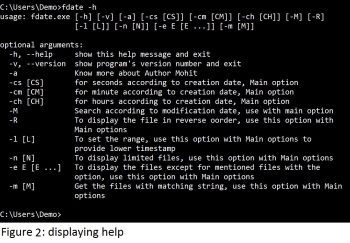This helps to show about author.

`Fdate`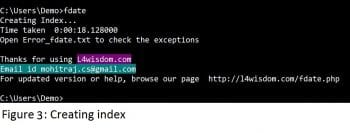Fdate without option creates a new index file. As shown in figure 3.

`Fdate -ch 1`

The above command displays the files which were created 1 hour ago. In figure 4 you can see the output. There are lots of files have been created by a user or system. But I want to get rid of system created files. I can use option -e show in figure 5.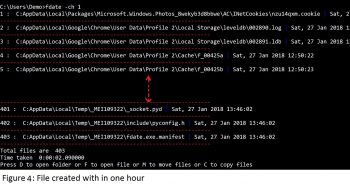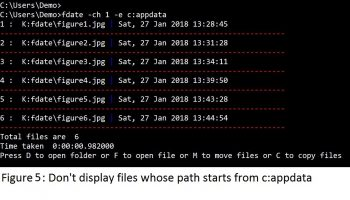By using -e option you supply a list of exception path which you don’t want to display.

Example

`Fdate -ch 1 -e c:app c:pycham`

It means we don’t to see the files whose path start from c:app or c:pycham.

Fdate -ch 1 -R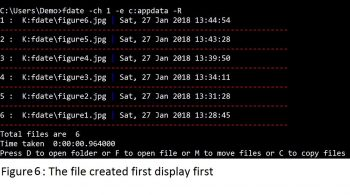With the -R command you can display the file in reverse order. As shown in figure 6.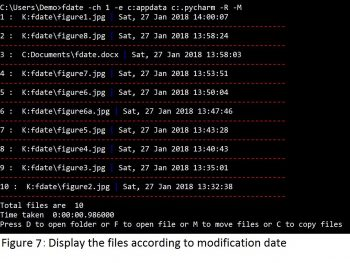`Fdate -ch 1 -M`

With the help of -M option, you can display the file according to modification date as shown in figure 7.

Consider you want to search a pdf file which you have downloaded 70 days ago. But you don’t remember the name. if you search files which were created 70*24 ago, it will be the huge number.

So, in order to tackle this problem, you can provide a lower limit of time means you can provide a time range with help of -l option.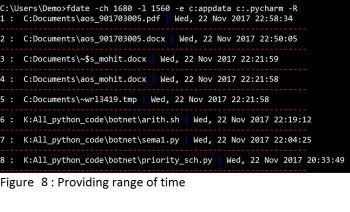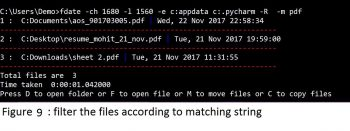I am going to display the file which were created 70 to 65 days ago. See the figure 8.

Still, there are lot of files, if you know the file is pdf then with the -m option you can provide the match. As shown in figure 9.

With help of -n you can display a limited number of files.

After displaying file, you can open the folder, open the file, move files or copy the files. As shown in figure 10. I selected 1,2,3 then I provided the e: drive which is my pen drive.

Consider You want to provide the exact date then you can use standard date format. With the help of -et option, you can provide the exact date as shown in the figure in 11. You can check the date format by using fdate -h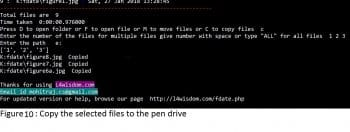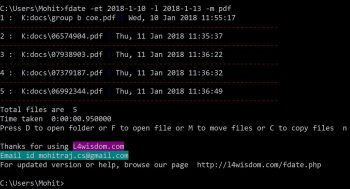You can see within a fraction of second I searched the files and copied the files.